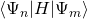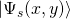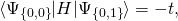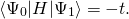# Retrieving the Hamiltonian from the Model in TBTK

Most recent TBTK release at the time of writing: v1.0.3
Updated to work with: v2.0.0

One of the most powerful aspects of TBTK is that it allows for models to be specified and properties to be extracted using physical indices such as {x, y, z, spin}. While this greatly simplifies the modeling process, the other side of the coin is the equally powerful means that are provided for method developers to write efficient general purpose code. In this post we will take a look at how a method developer typically would go about extracting the Hamiltonian from a Model and convert it to a format that is most efficient for the algorithm at hand.

## Model

Before moving on to the details of how to extract the Hamiltonian from the model, we here provide the code that sets up the example model that will be used in this tutorial.

//Parameters.
const int SIZE_X = 4;
const int SIZE_Y = 3;
double t = 1;

//Create the Model.
Model model;
for(unsigned int x = 0; x < SIZE_X; x++){
for(unsigned int y = 0; y < SIZE_Y; y++){
model << HoppingAmplitude(
4*t,
{x, y},
{x, y}
);
if(x + 1 < SIZE_X){
model << HoppingAmplitude(
-t,
{x + 1,	y},
{x,	y}
) + HC;
}
if(y + 1 < SIZE_Y){
model << HoppingAmplitude(
-t,
{x, y + 1},
{x, y}
) + HC;
}
}
}
model.construct();


For more details on the model specification itself, see for example Using TBTK to calculate the density of states (DOS) of a 1D, 2D, and 3D square lattice and Direct access to wave function amplitudes and eigenvalues in TBTK.

HoppingAmplitudes can generally be considered as matrix elements. While it from an algorithmic standpoint is very useful to considerto be a vector with a linear index, it is from an intuitive point of view more natural to work with states using a notation such as. In TBTK notation where both coordinates and indices necessarily are discrete, this can be encoded as {x, y, s}.

What the model.construct() call does for the model is to setup a one to one mapping between the physical indices and corresponding linear indices. That is, if for example {0, 0} is assigned the linear index 0, while {0, 1} is assigned the linear index 1, we can think of the following three expressions as equivalent1## HoppingAmplitudeSet

Inside the Model, the HoppingAmplitudes are stored in a structure called a HoppingAmplitudeSet, which can be considered a sparse matrix format that is tailored for quantum mechanics. It allows application developers to label the rows and columns of the matrix with physical indices, while simultaneously providing method developers with linear indices that runs from 0 to N-1, where N is the basis size.

We can get the HoppingAmplitudeSet from the Model and extract the basis size as follows.

//Get the HoppingAmplitudeSet from the Model
//and extract the basis size.
const HoppingAmplitudeSet &hoppingAmplitudeSet
= model.getHoppingAmplitudeSet();
unsigned int basisSize
= hoppingAmplitudeSet.getBasisSize();


## Set up the Hamiltonian

While the HoppingAmplitudeSet can be considered a sparse storage format for the HoppingAmplitudes, it is often not the most suitable format to use in a computationally demanding algorithm. Solution algorithms are usually where most of the execution time is spent, and the time they take to execute usually scales super linearly with the system size. It is therefore usually most reasonable to first convert the Hamiltonian to a format that is most suitable for the algorithm at hand. One of the core strengths of TBTK is that this conversion can be done very efficiently in a way that scale linearly with the system size.

In this example we will simply print the Hamiltonian once we have set it up. For simplicity we will therefore create a dense array in which we store the Hamiltonian.

//Initialize the Hamiltonian on a format most
//suitable for the algorithm at hand.
Array<complex<double>> hamiltonian(
{basisSize, basisSize},
0.
);


Next we iterate over all of the HoppingAmplitudes in the HoppingAmplitudeSet as follows.

//Iterate over the HoppingAmplitudes.
for(
HoppingAmplitudeSet::ConstIterator iterator
= hoppingAmplitudeSet.cbegin();
iterator != hoppingAmplitudeSet.cend();
++iterator
){
//Extract the amplitude and physical
//indices from the HoppingAmplitude.
complex<double> amplitude
= (*iterator).getAmplitude();
const Index &toIndex
= (*iterator).getToIndex();
const Index &fromIndex
= (*iterator).getFromIndex();

//Convert the physical indices to linear
//indices.
unsigned int row
= hoppingAmplitudeSet.getBasisIndex(
toIndex
);
unsigned int column
= hoppingAmplitudeSet.getBasisIndex(
fromIndex
);

//Write the amplitude to the Hamiltonian
//that will be used in this algorithm.
hamiltonian[{row, column}] += amplitude;
}


In line 10-15 we first extract the amplitude and the physical indices from the HoppingAmplitude. We then convert the physical indices to linear indices using hoppingAmplitudeSet.getBasisIndex(). Once this is done, we have the amplitude of the matrix element, as well as the linear row and column indices, and we can therefore write it to our dense array at the end of the loop body.2

Having set up the Hamiltonian we finally print it to verify that everything worked as expected.

//Print the Hamiltonian.
for(unsigned int row = 0; row < basisSize; row++){
for(unsigned int column = 0; column < basisSize; column++){
Streams::out << real(hamiltonian[{row, column}])
<< "\t";
}
Streams::out << "\n";
}


## Result

Executing the application we get the following print out.

 4 -1 0 -1 0 0 0 0 0 0 0 0 -1 4 -1 0 -1 0 0 0 0 0 0 0 0 -1 4 0 0 -1 0 0 0 0 0 0 -1 0 0 4 -1 0 -1 0 0 0 0 0 0 -1 0 -1 4 -1 0 -1 0 0 0 0 0 0 -1 0 -1 4 0 0 -1 0 0 0 0 0 0 -1 0 0 4 -1 0 -1 0 0 0 0 0 0 -1 0 -1 4 -1 0 -1 0 0 0 0 0 0 -1 0 -1 4 0 0 -1 0 0 0 0 0 0 -1 0 0 4 -1 0 0 0 0 0 0 0 0 -1 0 -1 4 -1 0 0 0 0 0 0 0 0 -1 0 -1 4

The validity of this output can be verified with the additional knowledge that in this specific case, the mapping between the physical indices and the linear Hilbert space indices is given by h = SIZE_Y*x + y.

## Full code

Full code is available in src/main.cpp in the project 2018_11_04 of the Second Tech code package. See the README for instructions on how to build and run.

1. When multiple HoppingAmplitudes with the same “to” and “from” Index is added to the Model, the matrix element is really the sum of all such HoppingAmplitudes.
2. Note that we in fact add the amplitude to the hamiltonian rather than setting it. This is to ensure that multiple HoppingAmplitudes with the same two indices all add up to the total matrix element. See also footnote 1.

## 2 Replies to “Retrieving the Hamiltonian from the Model in TBTK”

1.Frederick Moxley says:

Really like your work. Would be good to collaborate.

1.Kristofer Björnson says:

I’m glad to hear you like it!